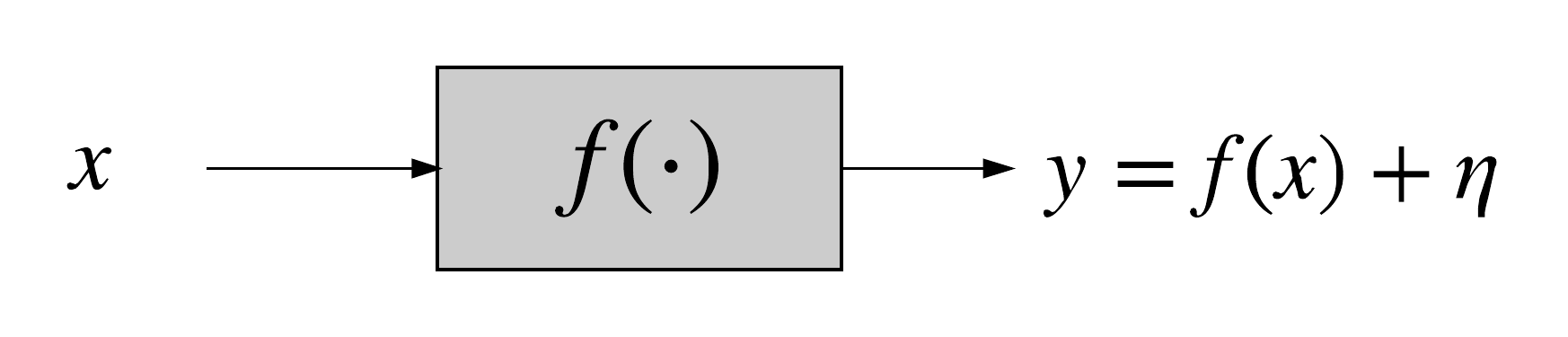A large class of problems can be solved by adaptively asking the right sequence of questions. By adaptive, we mean a strategy that uses all the collected information to guide the choice of the next action. A typical example is the binary search algorithm, that learns a threshold function with adaptive queries that, roughly, halve the residual uncertainty at each step.

A typical problem in this field is defined as follows: suppose there is an unknown function f:[0,1]^d \to \mathbb{R}, about which we only have some vague prior information (e.g., it lies in some function class \mathcal{F}). We can access this function through some local oracle queries. The most common oracle is the zeroth-order-oracle (ZOO)A noisy zeroth order oracle.

, that returns the noisy function value f(x) + \eta at any input x \in [0,1]^d. The goal, then, is to design an adaptive querying strategy to select a sequence of queries, x_1, x_2, \ldots, x_n, for the oracle in order to extract the most information about f to optimize some objective.

## Bayesian optimization

For the problem of Bayesian Optimization (BO), the objective is to find the maximizer (x^*) of f, or more formally, select the query points with small regret \mathcal{R}_n = \sum_{t=1}^n f(x^*) - f(x_t). Most BO algorithms, such as the widely used GP-UCB algorithm, proceed in round t \geq 1 in two steps GP-UCB, for example selects x_t by solving \max_{x \in [0,1]^d} \mu_t(x) + c \sigma_t(x), where \mu_t and \sigma_t are the posterior mean and variance of the fitted GP model; and c is some constant.

:

1. fit a surrogate Gaussian Process (GP) model to the previous observations, and
2. select the next query point, x_t, by solving an auxiliary optimization problem based on the surrogate model.

However, such algorithms suffer from a theory-practice gap: the theoretical analysis requires the above optimization problem to be solved exactly (or at least very accurately), which is often a \mathcal{O}(t^{2d}) operation, and thus not feasible in d \geq 5.

In my first paper S. Shekhar and T. Javidi, “Gaussian Process Bandits with Adaptive Discretization,” Electronic Journal of Statistics, 2018.

on this topic, I addressed this theory-practice gap by proposing a new algorithm that replaces the auxiliary maximization step with an inexpensive \mathcal{O}(td) operation. In addition to the significant computation gain, my algorithm also satisfies a tighter regret bound than GP-UCB, relying on new analysis techniques. Finally, the algorithm also performs well in practice, on the task of tuning the hyperparameters of a neural network.

In subsequent works, I have focused on obtaining a better understanding of the factors affecting the performance of BO algorithms. For instance it is known empirically, that incorporating noisy gradient information (when available) into a BO algorithm, often leads to an improvement in performance. In my AISTATS 2021 S. Shekhar and T. Javidi, “Significance of Gradient Information in Bayesian Optimization”, AISTATS 2021.

paper, I obtained a partial justification for this observation, by characterizing the amount of improvement that is possible with gradient based BO algorithms.

More recently, in my ICML 2022 S. Shekhar and T. Javidi, “Instance Dependent Regret Analysis of Kernelized Bandits”, ICML 2022.

paper, I obtained the first instance dependent regret bounds for the frequentist variant of BO problem (called kernelized bandits). This deviates from the existing analyses that study the worst case performance. Informally, my work answers the question: what aspect of a function f makes it easier or harder to optimize via noisy zeroth-order oracle queries?.

## Other Problems

The ideas developed in the above papers for Bayesian Optimization are quite general, and also extend to related problems. In my AISTATS 2019 S. Shekhar and T. Javidi, “Multi-Scale Gaussian Process Level Set Estimation”, AISTATS 2019.

paper, I developed a new algorithm for the problem of level-set estimation. This is a generalization of the optimization task, where, instead of finding just the optimizer x^*, we aim to find the entire region of the input space where the function takes values above a certain given threshold.

In my JSAIT, 2021 paper S. Shekhar, M. Ghavamzadeh and T. Javidi, “Active Learning for Classification with Abstention”, JSAIT 2021.

, I developed a near-optimal scheme for the problem of active learning with an abstain option. This problem models several safety-critical applications, such as medical diagnostics, where it is better to delay the decision, than to declare a wrong judgement.